# Relational Data Modeling - Association Table (Bridge, Cross)

An association table (bridge table) is a table that permits to implement:

Because most of database only support one-to-many relationships, it is necessary to implement other relationships physically via a third junction table.

An association table is also known as:

• cross-reference
• associative table
• Referencing table
• factless fact table

## Modeling

### One-to-One

To model a one-to-one relationship:

### Many-to-many

A Many-to-many relation is implemented via two one-to-many relationships:

• A → AB
• and B → AB.

In this case the logical primary key for AB is formed from the two foreign keys (i.e. copies of the primary keys of A and B).

The third table can be a fact table

#### Weighing Factor

The weighing factor is there mainly to distribute the dollar amounts evenly across the categories, where the sum of the parts cannot be greater than the total

Example:

For example, in a sport bet application:

• Bob has interests in Football and Baseball and spends 20,
• Alice has interests in Football, Soccer and Tennis and spends 30.

A revenue by breakdown cannot be…

``````Football: \$50
Baseball: \$20
Soccer:\$30
Tennis: \$30
```
```

because the total would 130 when in reality it's only 50.

The weighing factor will correct this logic. Ie the amount would be divide by the sports of interest for each person

The result would then be:

``````Football: 20/2 (Bob) + 30/3 (Alice) = \$20
Baseball: 20/2 (Bob) = \$10
Soccer: 30/3 (Alice) = \$10
Tennis: 30/3 (Alice) = \$10
```
```

with a total of 50

The problem with the weigh factor is that the user needs to know what the factor is for.

Recommended Pages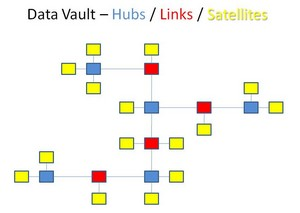A Link table in the data vault methodology is: transactions, associations, hierarchies, and re-definition of business terms. Every table which has more than one foreign key is a candidate...Data Warehousing - 34 Kimball Subsytems

This page takes back the Kimball Datawarehouse 34 Subsystem as a table of content and links them to a page on this website....Dimensional Data Modeling - Relationship

In a dimensional data model, you have mostly a one-to-many relationship between the dimension and the fact table between each level in a hierarchy man-to-many relationship are modeled with a bridge...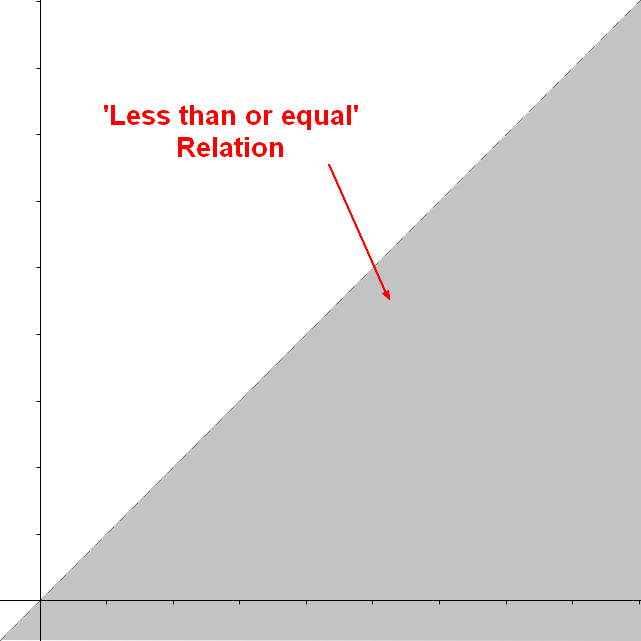Logical Data Modeling - Relationship Representation / Implementation / Visualisation

A relationship can be described/implemented by: a function a a a a A relationship can be represented as a function Example with a three element (ternary) relationship: A binary...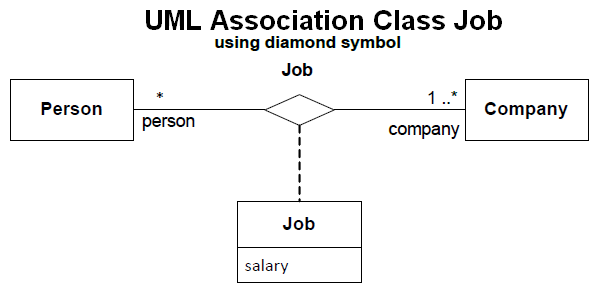Object - Association Class

An Association Class is a class that holds association information between two class. relational association table - 2.5.1 - page 207 for the figure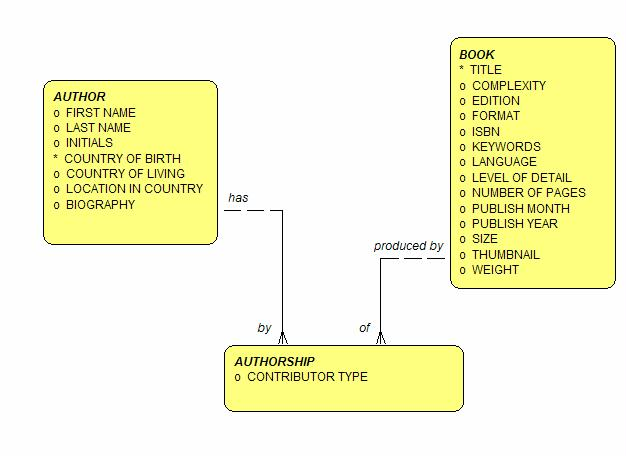Relational Data Modeling - Many-to-many Relationship (Two-way relationship)

A many-to-many relationship is a relationship (an m:n mapping) between two relation A and B in which : A may contain a parent row for which there are many children in B and vice versa. normalized...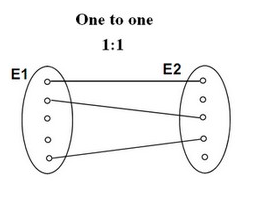Relational Data Modeling - One-to-One Relationship

A one-to-one relationship is a relational relationship that is equivalent to the set bijection relationship This relationship describe that one row from a relation point to exactly one row to the other...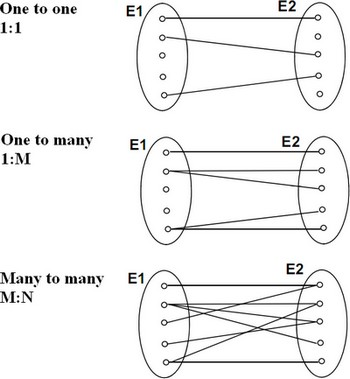Relational Data Modeling - Relationship

relationship in a relational model. relation (generally a table) an entity or a relationship cardinality define the type of relationships between two relations. third junction table (association...